# NCERT Solutions for Class 9 Maths Chapter 2 Exercise 2.2 – Polynomials

Download NCERT Solutions for Class 9 Maths Chapter 2 Exercise 2.2 – Polynomials. This Exercise contains 4 questions, for which detailed answers have been provided in this note. In case you are looking at studying the remaining Exercise for Class 9 for Maths NCERT solutions for Chapter 2 or other Chapters, you can click the link at the end of this Note.

### NCERT Solutions for Class 9 Maths Chapter 2 Exercise 2.2 – Polynomials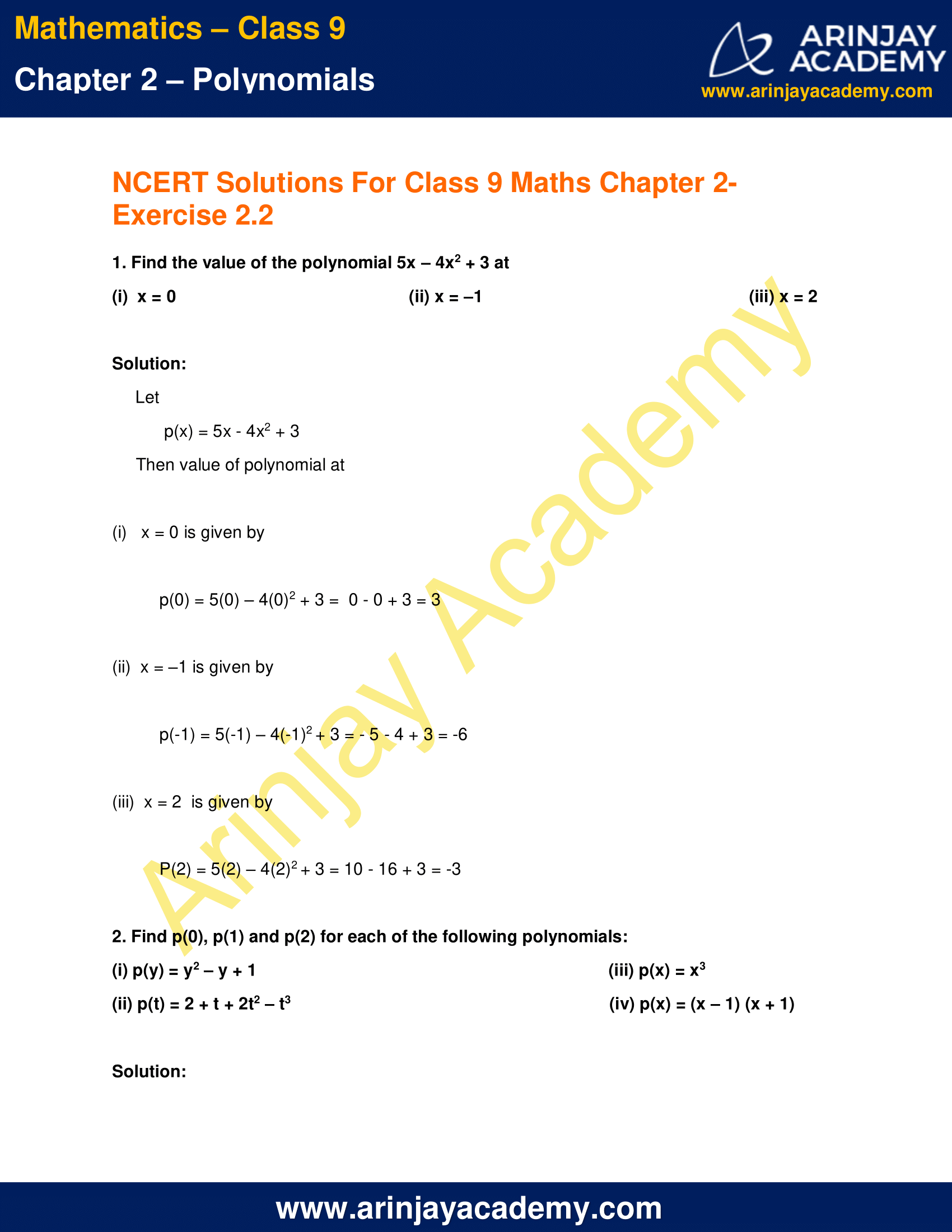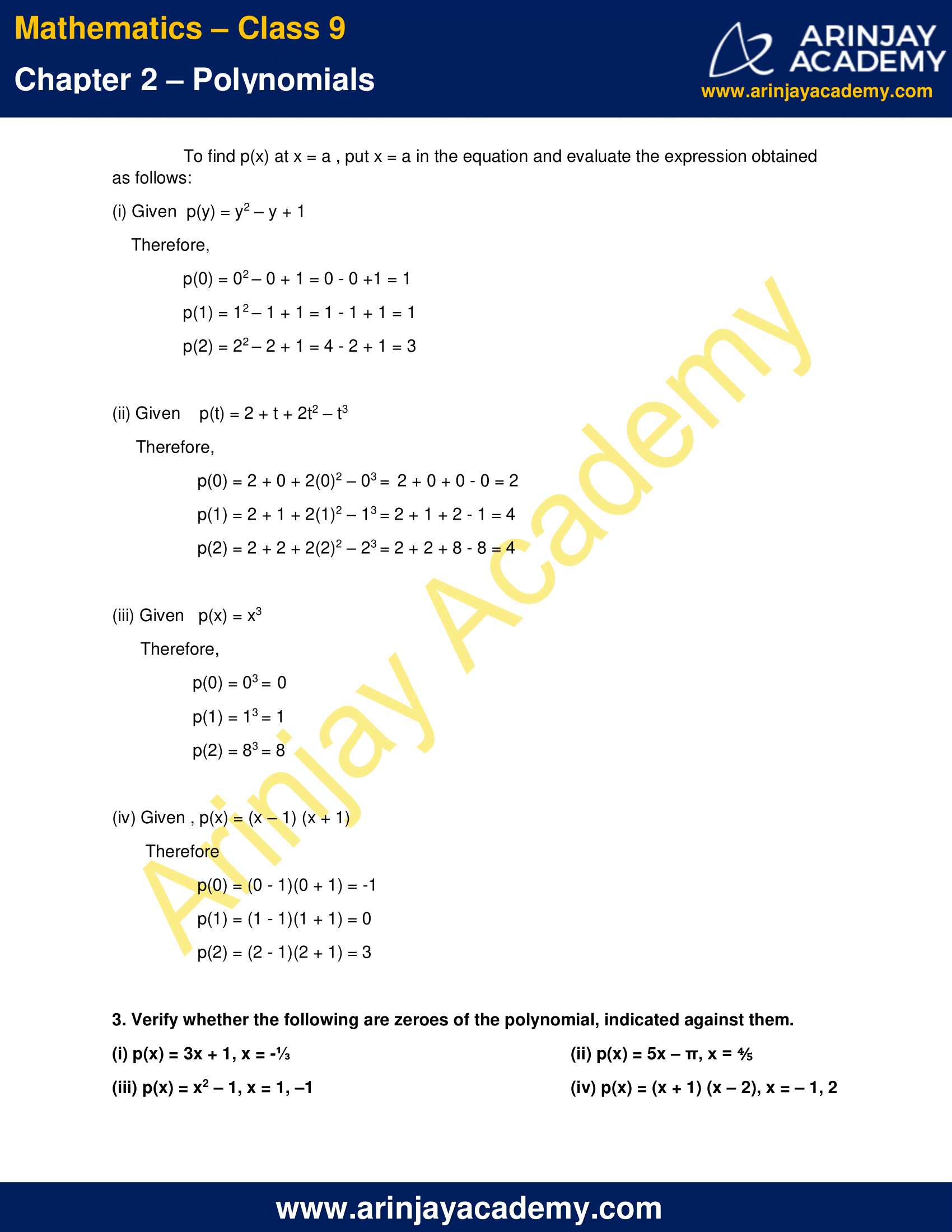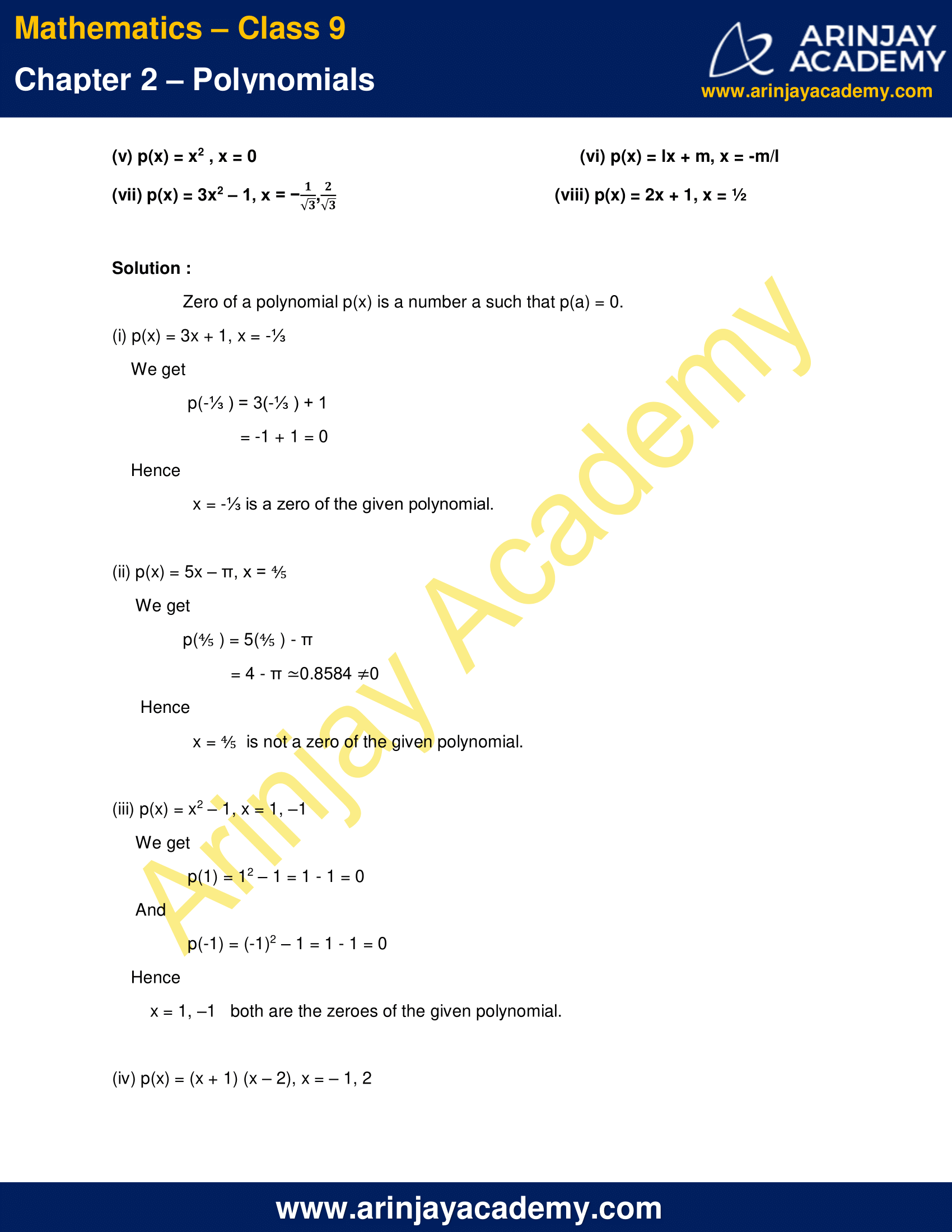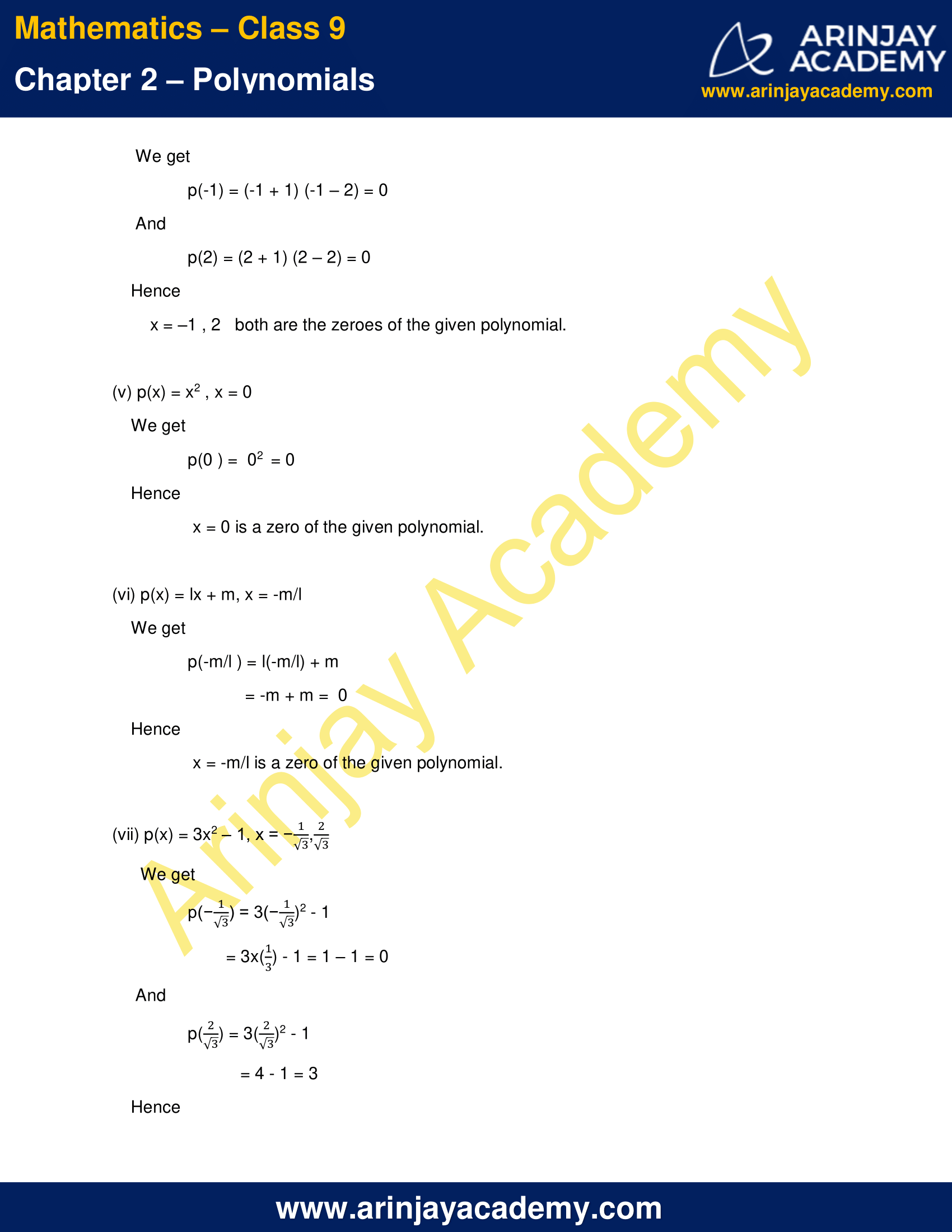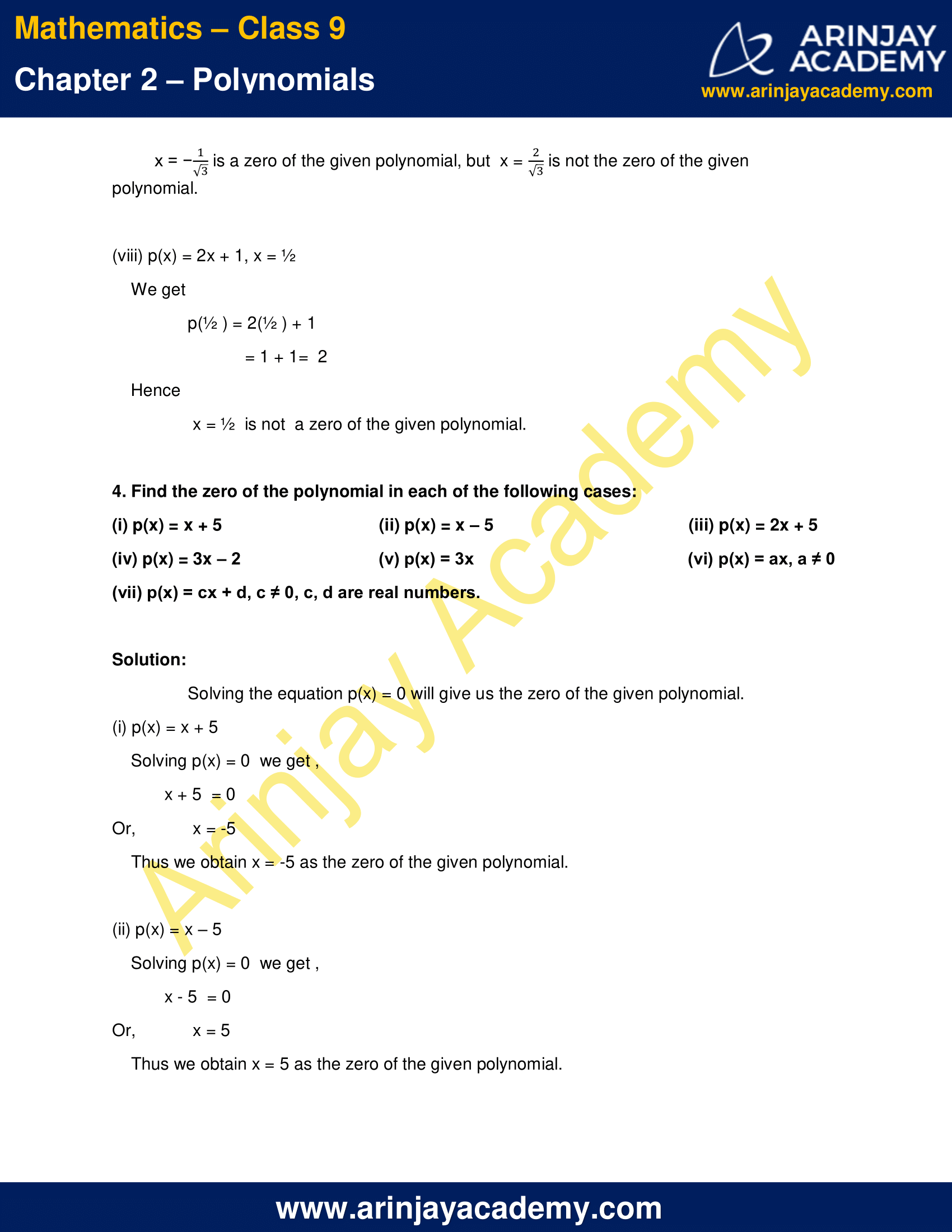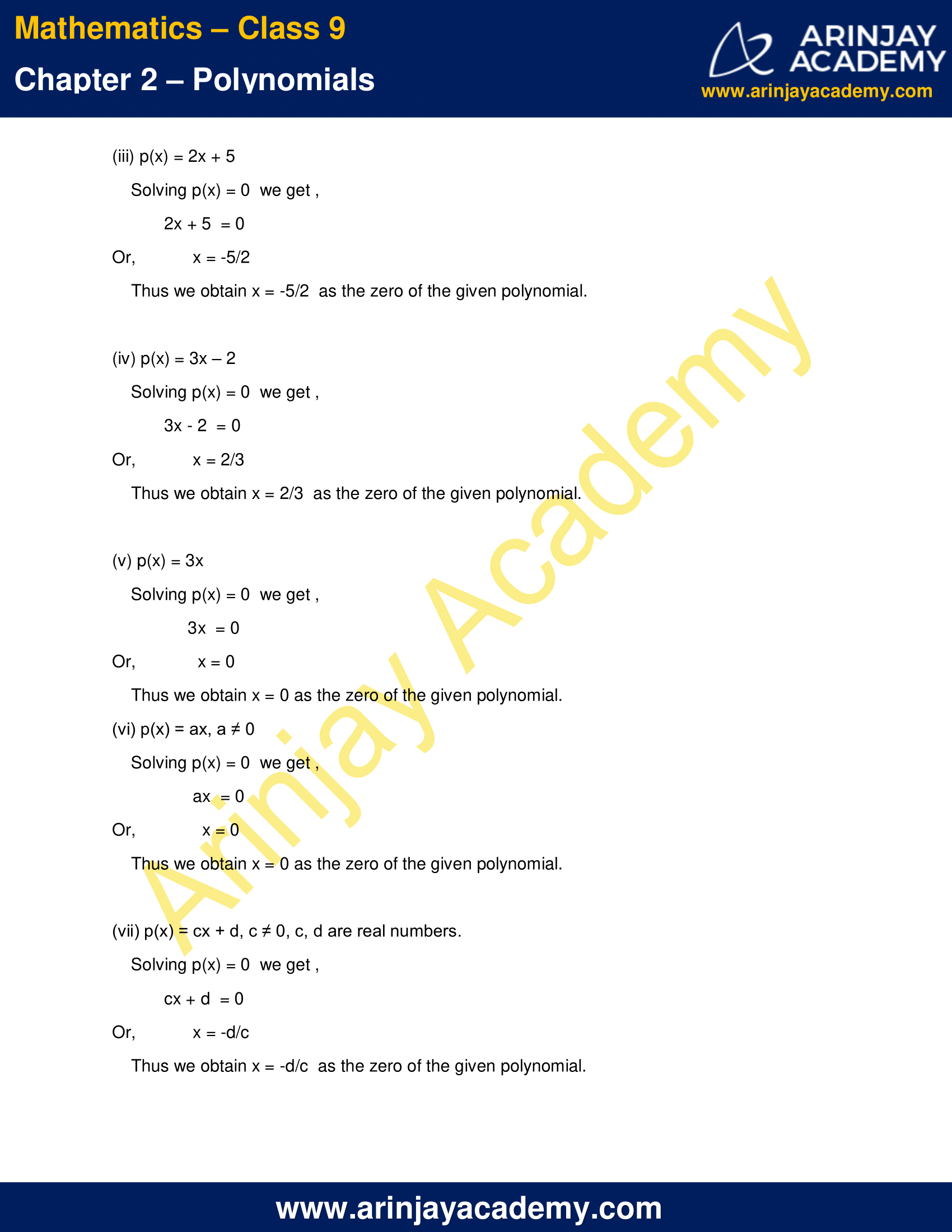NCERT Solutions for Class 9 Maths Chapter 2 Exercise 2.2 – Polynomials

1. Find the value of the polynomial 5x – 4x2 + 3 at

(i)  x = 0
(ii) x = –1
(iii) x = 2

Solution:

Let, p(x) = 5x – 4x2 + 3
Then value of polynomial at

(i)   x = 0 is given by
p(0) = 5(0) – 4(0)2 + 3 =  0 – 0 + 3 = 3

(ii)  x = –1 is given by
p(-1) = 5(-1) – 4(-1)2 + 3 = – 5 – 4 + 3 = -6

(iii)  x = 2  is given by
P(2) = 5(2) – 4(2)2 + 3 = 10 – 16 + 3 = -3

2. Find p(0), p(1) and p(2) for each of the following polynomials:

(i) p(y) = y2 – y + 1
(ii) p(t) = 2 + t + 2t2 – t3
(iii) p(x) = x3
(iv) p(x) = (x – 1) (x + 1)

Solution:

To find p(x) at x = a , put x = a in the equation and evaluate the expression obtained as follows:

(i) Given  p(y) = y2 – y + 1
Therefore,
p(0) = 02 – 0 + 1 = 0 – 0 +1 = 1
p(1) = 12 – 1 + 1 = 1 – 1 + 1 = 1
p(2) = 22 – 2 + 1 = 4 – 2 + 1 = 3

(ii) Given    p(t) = 2 + t + 2t2 – t3
Therefore,
p(0) = 2 + 0 + 2(0)2 – 03 =  2 + 0 + 0 – 0 = 2
p(1) = 2 + 1 + 2(1)2 – 13 = 2 + 1 + 2 – 1 = 4
p(2) = 2 + 2 + 2(2)2 – 23 = 2 + 2 + 8 – 8 = 4

(iii) Given   p(x) = x3
Therefore,
p(0) = 03 =  0
p(1) = 13 = 1
p(2) = 83 = 8

(iv) Given , p(x) = (x – 1) (x + 1)
Therefore,
p(0) = (0 – 1)(0 + 1) = -1
p(1) = (1 – 1)(1 + 1) = 0
p(2) = (2 – 1)(2 + 1) = 3

3. Verify whether the following are zeroes of the polynomial, indicated against them.

(i) p(x) = 3x + 1, x = (-1/3)
(ii) p(x) = 5x – π, x = (4/5)
(iii) p(x) = x2 – 1, x = 1, –1
(iv) p(x) = (x + 1) (x – 2), x = – 1, 2
(v) p(x) = x2 , x = 0
(vi) p(x) = lx + m, x = -m/l
(vii) p(x) = 3x2 – 1, x = −(1/√3), (2/√3)
(viii) p(x) = 2x + 1, x = 1/2

Solution :

Zero of a polynomial p(x) is a number a such that p(a) = 0.

(i) p(x) = 3x + 1, x = (-1/3)
We get,
p(1/3) = 3 x (-1/3) + 1
= -1 + 1 = 0
Hence, x = (-1/3) is a zero of the given polynomial.

(ii) p(x) = 5x – π, x = 4/5
We get,
p(4/5) = 5 x (4/5) – π
= 4 – π ≈ 0.8584 ≠ 0
Hence, x = (4/5) is not a zero of the given polynomial.

(iii) p(x) = x2 – 1, x = 1, –1
We get,
p(1) = 12 – 1 = 1 – 1 = 0
p(-1) = (-1)2 – 1 = 1 – 1 = 0
Hence, x = 1, –1   both are the zeroes of the given polynomial.

(iv) p(x) = (x + 1) (x – 2), x = – 1, 2
We get,
p(-1) = (-1 + 1) (-1 – 2) = 0
p(2) = (2 + 1) (2 – 2) = 0
Hence, x = –1 , 2   both are the zeroes of the given polynomial.

(v) p(x) = x2 , x = 0
We get,
p(0 ) =  02  = 0
Hence, x = 0 is a zero of the given polynomial.

(vi) p(x) = lx + m, x = (-m/l)
We get
p(-m/l ) = l(-m/l) + m
= -m + m =  0
Hence, x = -m/l is a zero of the given polynomial.

(vii) p(x) = 3x2 – 1, x = −(1/√3), (2/√3)
We get,
p[−(1/√3)] = 3(−1/√3)2 – 1
= 3 x (1/3) – 1 = 1 – 1 = 0
p(2/√3) = 3(2/√3)2 – 1
= 4 – 1 = 3
Hence, x = −(1/√3) is a zero of the given polynomial, but  x = 2/√3  is not the zero of the given polynomial.

(viii) p(x) = 2x + 1, x = ½
We get,
p(½ ) = 2 x (1/2) + 1
= 1 + 1=  2
Hence, x = (1/2)  is not  a zero of the given polynomial.

4. Find the zero of the polynomial in each of the following cases:

(i) p(x) = x + 5
(ii) p(x) = x – 5
(iii) p(x) = 2x + 5
(iv) p(x) = 3x – 2
(v) p(x) = 3x
(vi) p(x) = ax, a ≠ 0
(vii) p(x) = cx + d, c ≠ 0, c, d are real numbers.

Solution:

Solving the equation p(x) = 0 will give us the zero of the given polynomial.

(i) p(x) = x + 5
Solving p(x) = 0  we get ,
x + 5  = 0
Or, x = -5
Thus we obtain x = -5 as the zero of the given polynomial.

(ii) p(x) = x – 5
Solving p(x) = 0  we get ,
x – 5  = 0
Or, x = 5
Thus we obtain x = 5 as the zero of the given polynomial.

(iii) p(x) = 2x + 5
Solving p(x) = 0  we get ,
2x + 5  = 0
Or, x = (-5/2)
Thus we obtain x = (-5/2) as the zero of the given polynomial.

(iv) p(x) = 3x – 2
Solving p(x) = 0  we get ,
3x – 2  = 0
Or, x = (2/3)
Thus we obtain x = 2/3  as the zero of the given polynomial.

(v) p(x) = 3x
Solving p(x) = 0  we get ,
3x  = 0
Or, x = 0
Thus we obtain x = 0 as the zero of the given polynomial.

(vi) p(x) = ax, a ≠ 0
Solving p(x) = 0  we get ,
ax = 0
Or,  x = 0
Thus we obtain x = 0 as the zero of the given polynomial.

(vii) p(x) = cx + d, c ≠ 0, c, d are real numbers.
Solving p(x) = 0  we get ,
cx + d  = 0
Or,  x = (-d/c)
Thus we obtain x = (-d/c) as the zero of the given polynomial.

NCERT Solutions for Class 9 Maths Chapter 2 Exercise 2.2 – Polynomials, has been designed by the NCERT to test the knowledge of the student on the topic – Zeroes of a Polynomial

The next Exercise for NCERT Solutions for Class 9 Maths Chapter 2 Exercise 2.3 – Number System can be accessed by clicking here.

Download NCERT Solutions for Class 9 Maths Chapter 2 Exercise 2.2 – Polynomials